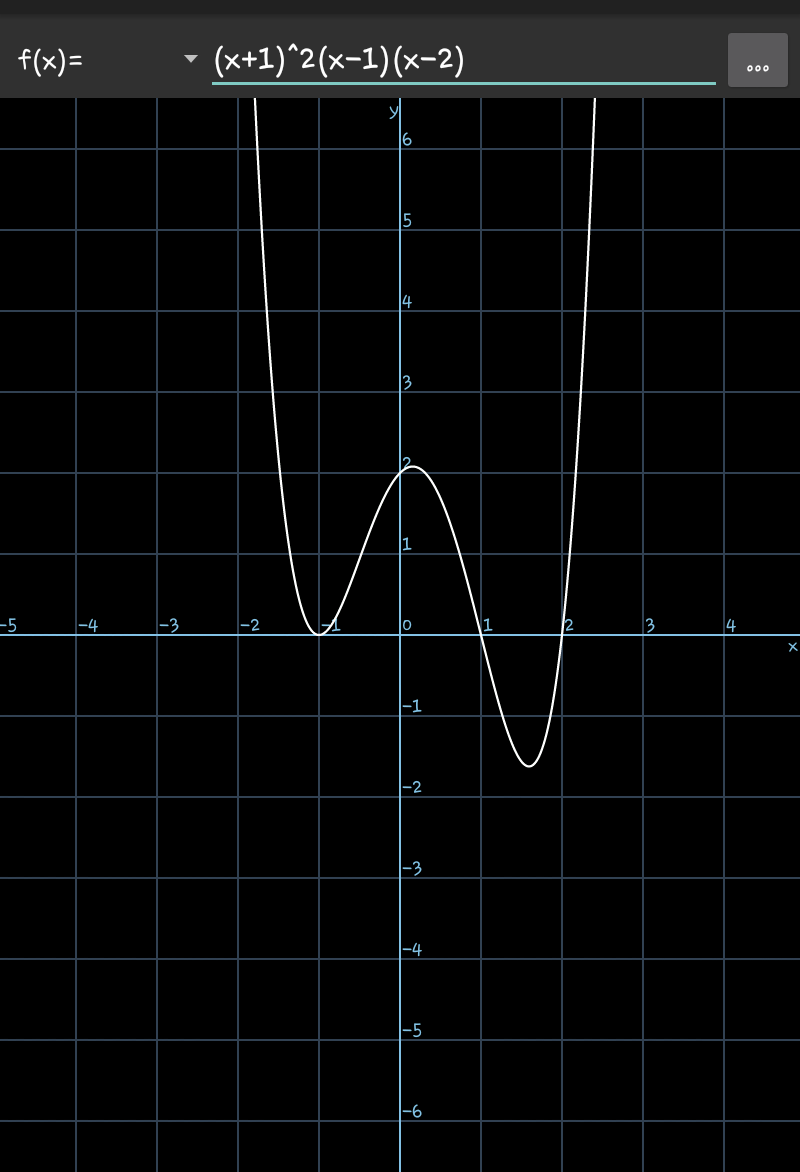# Doubt from Continuity and differentiability

f(x) is non-differentiable for x = 0, 2?

Sin |x| will be non-differentiable at x=0, and the rest of function will be differentiable, so f(x) will overall be non-differentiable.

At x=1, sine function is differentiable but the |x²-x-2| will be be differentiable because x²-1 will be equal to zero, so LHD = RHD = 0 (for algebraic part of f(x))

Similarly, you can check at x = 2, f(x) will be non differentiable.

From graph it is clear that sin(modx) not differentiable at x=0
F(x)=g(x)+h(x) g(x)=algebric function differentiable
h(x)=sin(modx) not differentiable
So F(x) not differentiable at x=0Sin|x| is not differentiable at 0 and and another portion of function is differentiable at point x=1 so overall it's non differentiable at x=0